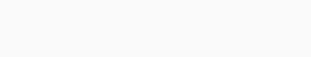In this section we will learn about QP(Quadratic Programming). It will help you to understand more about optimization and you will be able to use it more precisely. We will walk you through some stuff with examples and also show you some examples to make things easy for you. So let’s begin.

Mathematical optimization problems involving quadratic functions can be solved using quadratic programming (QP). A multivariate quadratic function subject to linear constraints is optimized (minimized or maximized). In quadratic programming, an objective function is maximized (or minimized) under one or more constraints. Statistical work occasionally uses the technique in operations research.

The term “programming” refers to a formal procedure for solving mathematical problems. As a result, some practitioners prefer the term “optimization” – for example, “quadratic optimization” – to avoid confusion with the more recent concept of “computer programming.” Nonlinear programming is a type of quadratic programming.

There can be bilinear or up to second order polynomial terms in the objective function, and the constraints can be linear and equalities or inequalities. Among the many applications of QP are image and signal processing, financial portfolio optimization, least-squares regression, chemical plant scheduling, and sequential quadratic programming, a method for resolving nonlinear programming problems of greater complexity.Example

This section shows how to run the data presented in the example given above using quadratic programming. The data are contained in the QP database. Here is the specification of the problem.## Application

• Model predictive control

Chemical engineering also uses quadratic programming. Model predictive control (MPC) helps manage production in chemical plants by dictating production in each batch. Even though MPC models are often formulated as linear programs because they are more robust, stable, and easier to solve, quadratic programming is sometimes used as well. Di Ruscio used quadratic programming in an MPC algorithm for a thermomechanical pulping process as an example of its utility.

• Least squares

One of the most common types of regression is least squares regression, which minimizes the sum of squares of the difference between data points and a proposed fit. Most linear regression methods are more rigid than quadratic optimization when it comes to performing least squares regression.

## Code

				
%use s2


				
/**
* example 13.1 in
* Andreas Antoniou, Wu-Sheng Lu, "Algorithm 13.1, Quadratic and Convex
* Programming," Practical Optimization: Algorithms and Engineering
* Applications.
*
* @throws Exception
*/

println("Solving an QP problem with only equality constraints")

//construct the QP problem with only equality constraints
val H: DenseMatrix = DenseMatrix(
arrayOf(
doubleArrayOf(1.0, 0.0, 0.0),
doubleArrayOf(0.0, 1.0, 0.0),
doubleArrayOf(0.0, 0.0, 0.0)
)
)

val p: DenseVector = DenseVector(2.0, 1.0, -1.0)
println("minimizing:")
println(f)

// equality constraints
val A: DenseMatrix = DenseMatrix(
arrayOf(
doubleArrayOf(0.0, 1.0, 1.0)
))
val b: DenseVector = DenseVector(1.0)
val Aeq = LinearEqualityConstraints(A, b)

// solve a QP problem with only equality constraints
val soln = QPSimpleMinimizer.solve(f, Aeq)
val x: Vector = soln.minimizer()
val fx: Double = f.evaluate(x)
println(String.format("f(%s) = %f%n", x, fx))
println(String.format("is unique = %b%n", soln.isUnique()))/**
* example 16.4 in Jorge Nocedal, Stephen Wright
*
* There is a detailed trace (for debugging) on p. 475.
*/

println("Solving an QP problem")

val H: Matrix = DenseMatrix(
arrayOf(
doubleArrayOf(2.0, 0.0),
doubleArrayOf(0.0, 2.0),
)
)

val p: Vector = DenseVector(-2.0, -5.0)

// construct the linear inequality constraints

val A: Matrix = DenseMatrix(
arrayOf(
doubleArrayOf(1.0, -2.0),
doubleArrayOf(-1.0, -2.0),
doubleArrayOf(-1.0, 2.0),
doubleArrayOf(1.0, 0.0),
doubleArrayOf(0.0, 1.0),
)
)

val b: Vector = DenseVector(-2.0, -6.0, -2.0, 0.0, 0.0)
val greater = LinearGreaterThanConstraints(A, b)// x &gt;= 0
// construct the QP problem
val problem = QPProblem(f, null, greater)

// construct a primal active set solver
val epsion: Double = Math.sqrt(PrecisionUtils.autoEpsilon(problem.f().Hessian()))
val solver1 = QPPrimalActiveSetMinimizer(
epsion, // precision
Integer.MAX_VALUE // max number of iterations
)
// solve the QP problem using the primal active set method
val solution1: QPPrimalActiveSetMinimizer.Solution = solver1.solve(problem)
solution1.search(DenseVector(2.0, 0.0))
// print out the solution
println("minimizer = " + solution1.minimizer().minimizer())
println("minimum = " + solution1.minimum())val H: Matrix = DenseMatrix(
arrayOf(
doubleArrayOf(2.0, 0.0),
doubleArrayOf(0.0, 2.0),
)
)

val p: Vector = DenseVector(-2.0, -5.0)

// construct the linear inequality constraints

val A: Matrix = DenseMatrix(
arrayOf(
doubleArrayOf(1.0, -2.0),
doubleArrayOf(-1.0, -2.0),
doubleArrayOf(-1.0, 2.0),
doubleArrayOf(1.0, 0.0),
doubleArrayOf(0.0, 1.0),
)
)

val b: Vector = DenseVector(-2.0, -6.0, -2.0, 0.0, 0.0)
val greater = LinearGreaterThanConstraints(A, b)// x &gt;= 0
// construct the QP problem
val problem = QPProblem(f, null, greater)

// construct a dual active set solver
val epsion: Double = Math.sqrt(PrecisionUtils.autoEpsilon(problem.f().Hessian()))
// solve the QP problem using the dual active set method
val solver2 = QPDualActiveSetMinimizer(
epsion, // precision
Integer.MAX_VALUE) // max number of iterations
val solution2: QPDualActiveSetMinimizer.Solution = solver2.solve(problem)
solution2.search()
// print out the solution
println("minimizer = " + solution2.minimizer().minimizer())
println("minimum = " + solution2.minimum())## Conclusion

It is a simple form of non-linear programming that can accurately model many real-world systems, notably those whose variables are dependent on two variables, since quadratic programming was developed in the 1950s. When the objective function is convex, these problems are straightforward to solve. There are applications for QP in finance, various types of computer systems, statistics, chemical production, and in algorithms to solve more complex natural language processing problems.